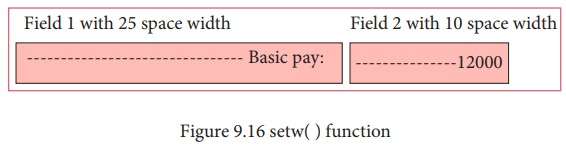Home | | Computer Science 11th std | C++: Formatting Output

# C++: Formatting Output

Formatting output is very important in the development of output screens for easy reading and understanding.

Formatting Output:

Formatting output is very important in the development of output screens for easy reading and understanding. Manipulators are used to format the output of any C++ program. Manipulators are functions specifically designed to use with the insertion (<<) and extraction(>>) operators.

C++ offers several input and output manipulators for formatting. Commonly used manipulators are: endl, setw, setfill, setprecision and setf. In order to use these manipulators, you should include the appropriate header file. endl manipulator is a member of iostream header file. setw, setfill, setprecision and setf manipulators are members of iomanip header file.

## endl (End the Line)

endl is used as a line feeder in C++. It can be used as an alternate to ‘\n’. In other words, endl inserts a new line and then makes the cursor to point to the beginning of the next line. There is a difference between endl and ‘\n’, even though they are performing similar tasks.

• endl – Inserts a new line and flushes the buffer (Flush means – clean)

• ‘\n’ - Inserts only a new line.

### Example:

cout << "\n The value of num = " << num;

cout << "The value of num = " << num <<end;

Both these statements display the same output.

## setw ( )

setw manipulator sets the width of the field assigned for the output. The field width determines the minimum number of characters to be written in output.

### Syntax:

setw(number of characters)

## Illustration 9.10: Program to Calculate Net Salary

#include <iostream>

#include <iomanip>

using namespace std;

int main()

{

float basic, da, hra, gpf, tax, gross, np;

char name;

cout << "\n Enter Basic Pay: ";

cin >> basic;

cout << "\n Enter D.A : ";

cin >> da;

cout << "\n Enter H.R.A: ";

cin >> hra;

gross = basic+da+hra; // sum of basic, da nad hra

gpf = (basic+da) * 0.10; // 10% 0f basic and da

tax = gross * 0.10; //10% of gross pay

np = gross - (gpf+tax); //netpay = earnings - deductions

cout << setw(25) << "Basic Pay : " << setw(10)<< basic<< endl;

cout << setw(25) << "Dearness Allowance : "<< setw(10)<<da<< endl;

cout<<setw(25)<<"House Rent Allowance : "<<setw(10)<< hra<<endl;

cout << setw(25) << "Gross Pay : " << setw(10) << gross << endl;

cout << setw(25) << "G.P.F : " << setw(10) << gpf << endl;

cout << setw(25) << "Income Tax : " << setw(10)<< tax << endl;

cout << setw(25) << "Net Pay : " << setw(10) << np << endl;

}

### The output will be,

Enter Basic Pay: 12000

Enter D.A : 1250

Enter H.R.A : 1450

Basic Pay : 12000

Dearness Allowance : 1250

House Rent Allowance : 1450

Gross Pay : 14700

G.P.F : 1325

Income Tax : 1470

Net Pay : 11905

(HOT: Try to make multiple output statements as a single cout statement)

In the above program, every output statement has two setw( ) manipulators; first setw creates a filed with 25 spaces and second setw(10) creates another field with 10 spaces. When you represent a value to these fields, it will show the value within the field from right to left.In field1 and field 2, the string “Basic Pay: ” and the value of basic pay are shown as given in Figure 9.16 below.## setfill ( )

This manipulator is usually used after setw. If the presented value does not entirely fill the given width, then the specified character in the setfill argument is used for filling the empty fields.

### Syntax:

setfill (character);

### Example:

cout << "\n H.R.A : " << setw(10) << setfill (0) << hra;

In the above code, setw creates a field to show the presented value, setfill is used to fill un-occupied spaces with 0 (zero).

For example, if you assign 1200 to hra, setw accommodates 1200 in a field of width 10 from right to left and setfill fills 0 in the remaining 6 spaces that are in the beginning. The output will be, 0000001200.

## setprecision ( )

This is used to display numbers with fractions in specific number of digits.

### Syntax:

setprecision (number of digits);

### Example:

#include <iostream>

#include <iomanip>

using namespace std;

int main()

{

float hra = 1200.123;

cout << setprecision (5) << hra;

}

In the above code, the given value 1200.123 will be displayed in 5 digits including fractions. So, the output will be 1200.1

setprecision ( ) prints the values from left to right. For the above code, first, it will take 4 digits and then prints one digit from fractional portion.

setprecision can also be used to set the number of decimal places to be displayed. In order to do this task, you will have to set an ios flag within setf() manipulator. This may be used in two forms: (i) fixed and (ii) scientific

These two forms are used when the keywords fixed or scientific are appropriately used before the setprecision manipulator.

### Example:

#include <iostream>

#include <iomanip>

using namespace std;

int main()

{

cout.setf(ios::fixed);

cout << setprecision(2)<<0.1;

}

In the above program, ios flag is set to fixed type; it prints the floating point number in fixed notation. So, the output will be, 0.10

## cout.setf(ios::scientific);

cout << setprecision(2) << 0.1;

In the above statements, ios flag is set to scientific type; it will print the floating point number in scientific notation. So, the output will be, 1.00e-001

Study Material, Lecturing Notes, Assignment, Reference, Wiki description explanation, brief detail
11th Computer Science : Chapter 9 : Introduction to C++ : C++: Formatting Output |